# RD Sharma Solutions for Class 8 Maths Chapter 5 - Playing with Numbers Exercise 5.2

Download free pdf of RD Sharma Class 8 Maths Chapter 5 Playing with Numbers. Experts at BYJU’S have designed these solutions in a very clear manner that helps students solve problems in the most efficient possible ways. Securing good marks has become much easier by the usage of Solutions of RD Sharma Class 8 Maths. In Exercise 5.2 we shall discuss problems based on the test of divisibility by 10, 5, 2, 9, 3, 6, 11, 4. Students can refer and download RD Sharma Class 8 Solutions for Maths from the links provided below.

## Download the pdf of RD Sharma Solutions For Class 8 Maths Chapter 5 Playing with Numbers Exercise 5.2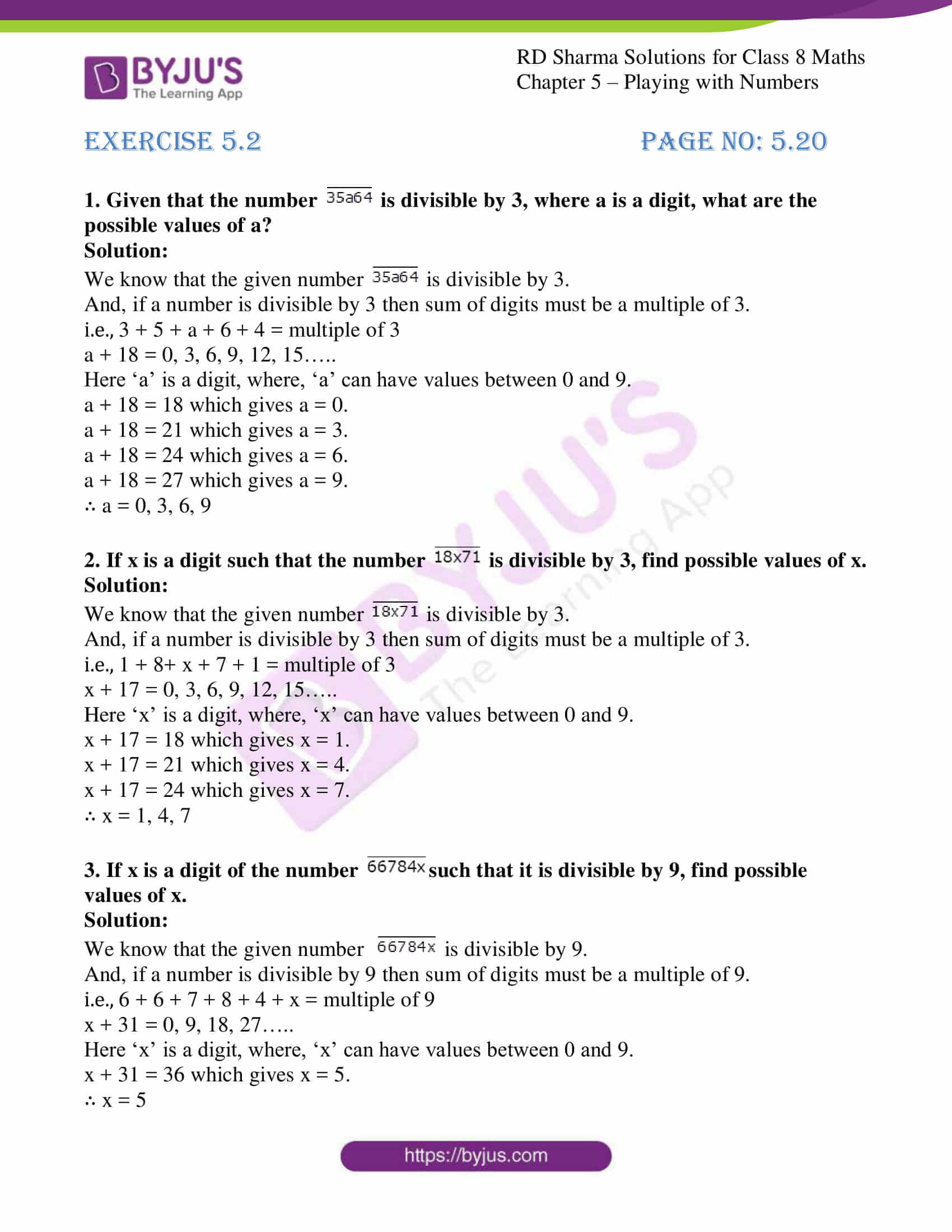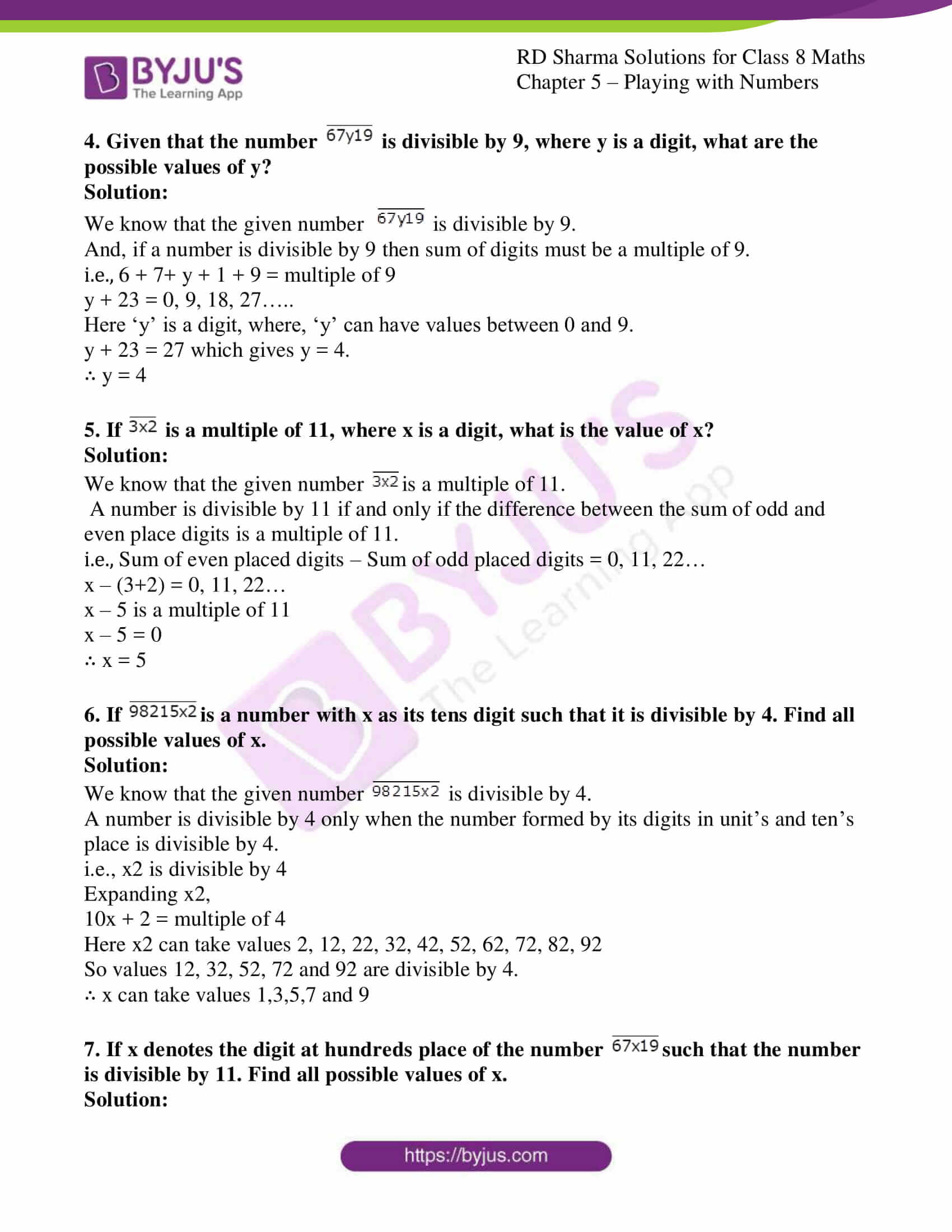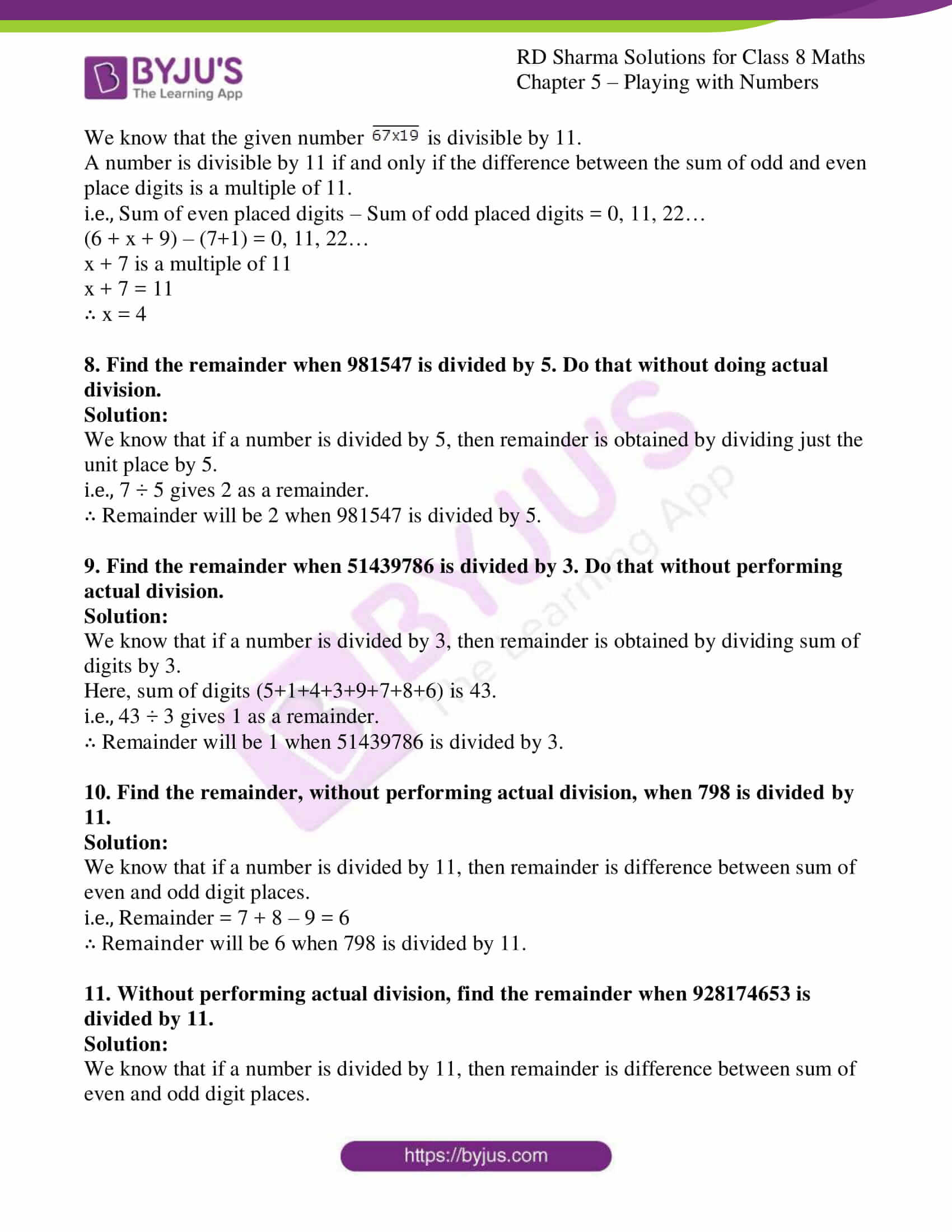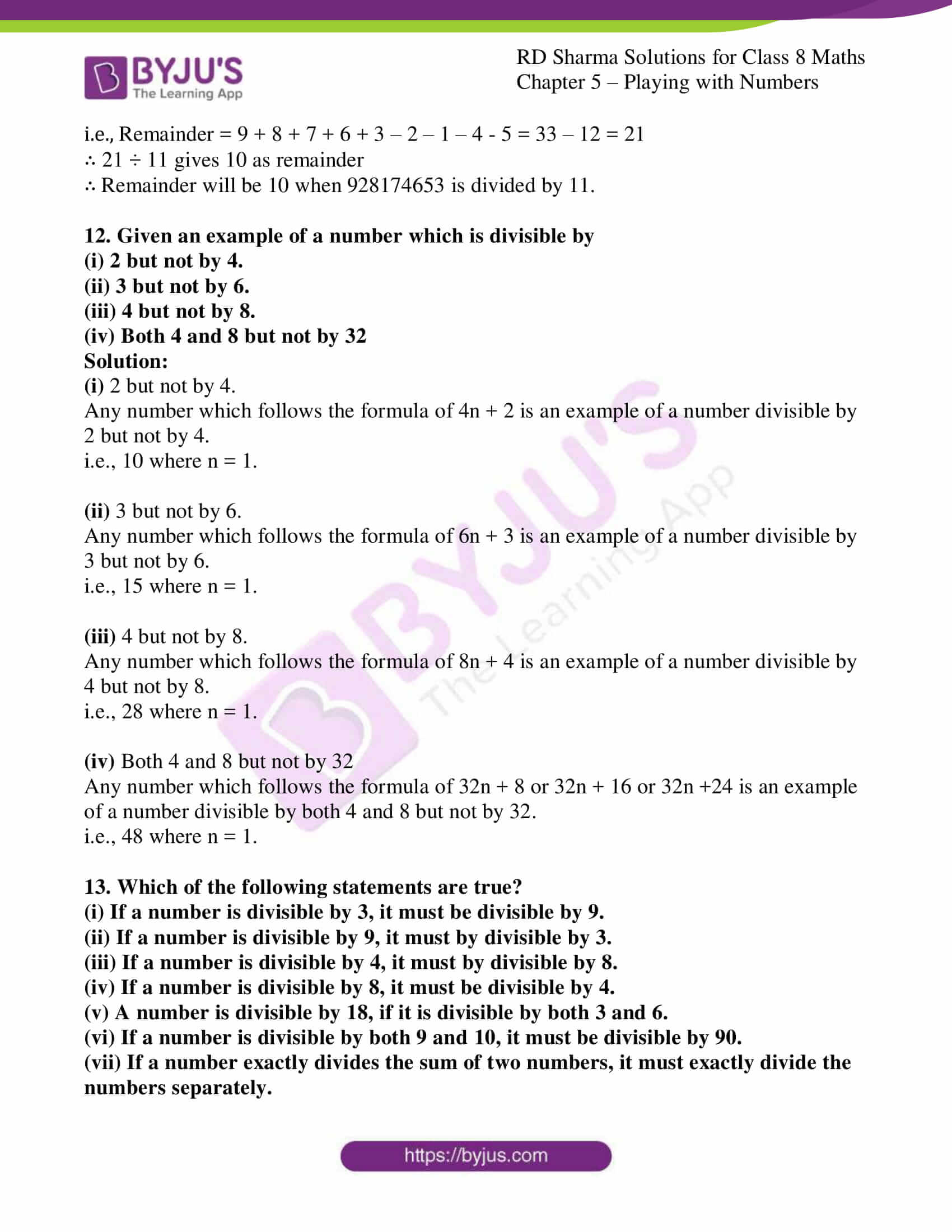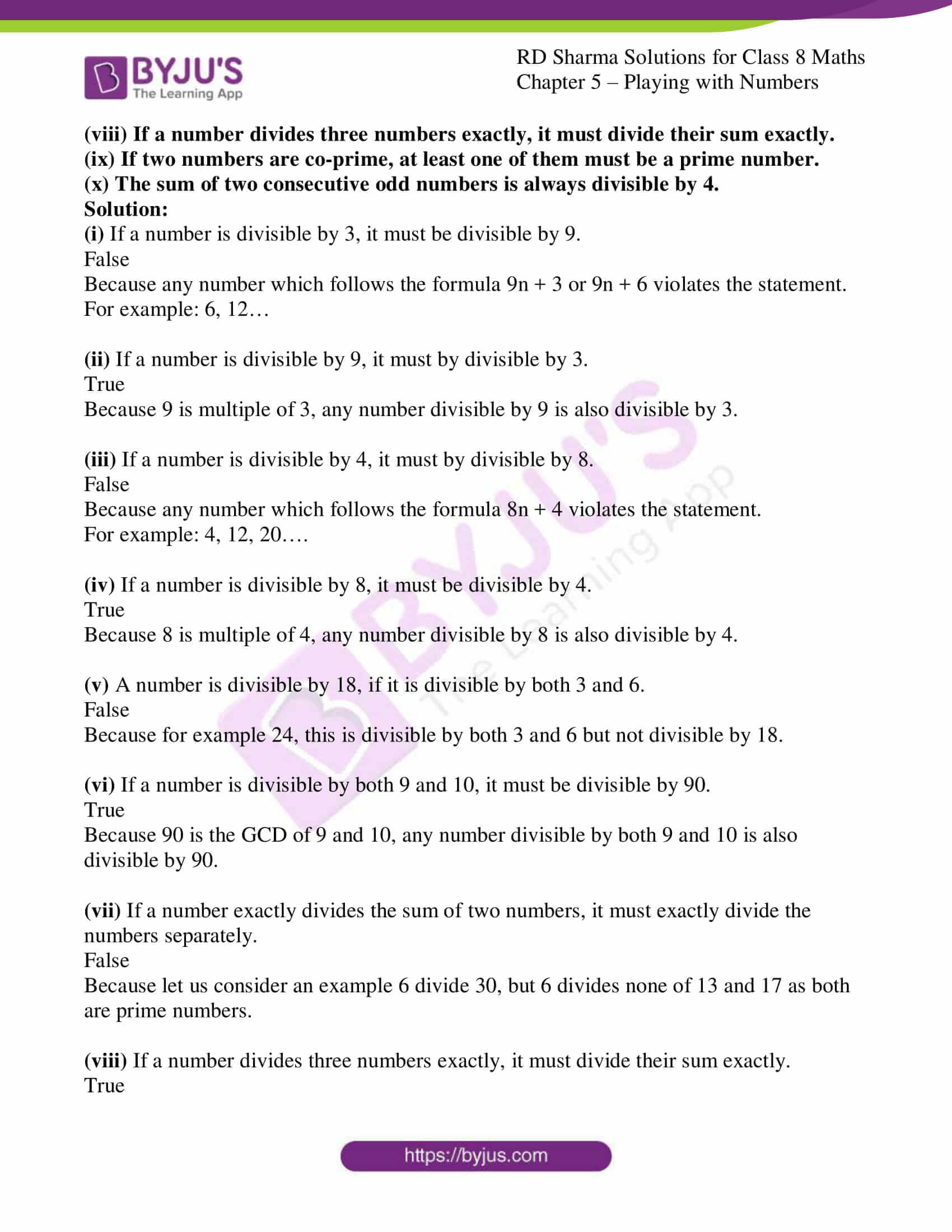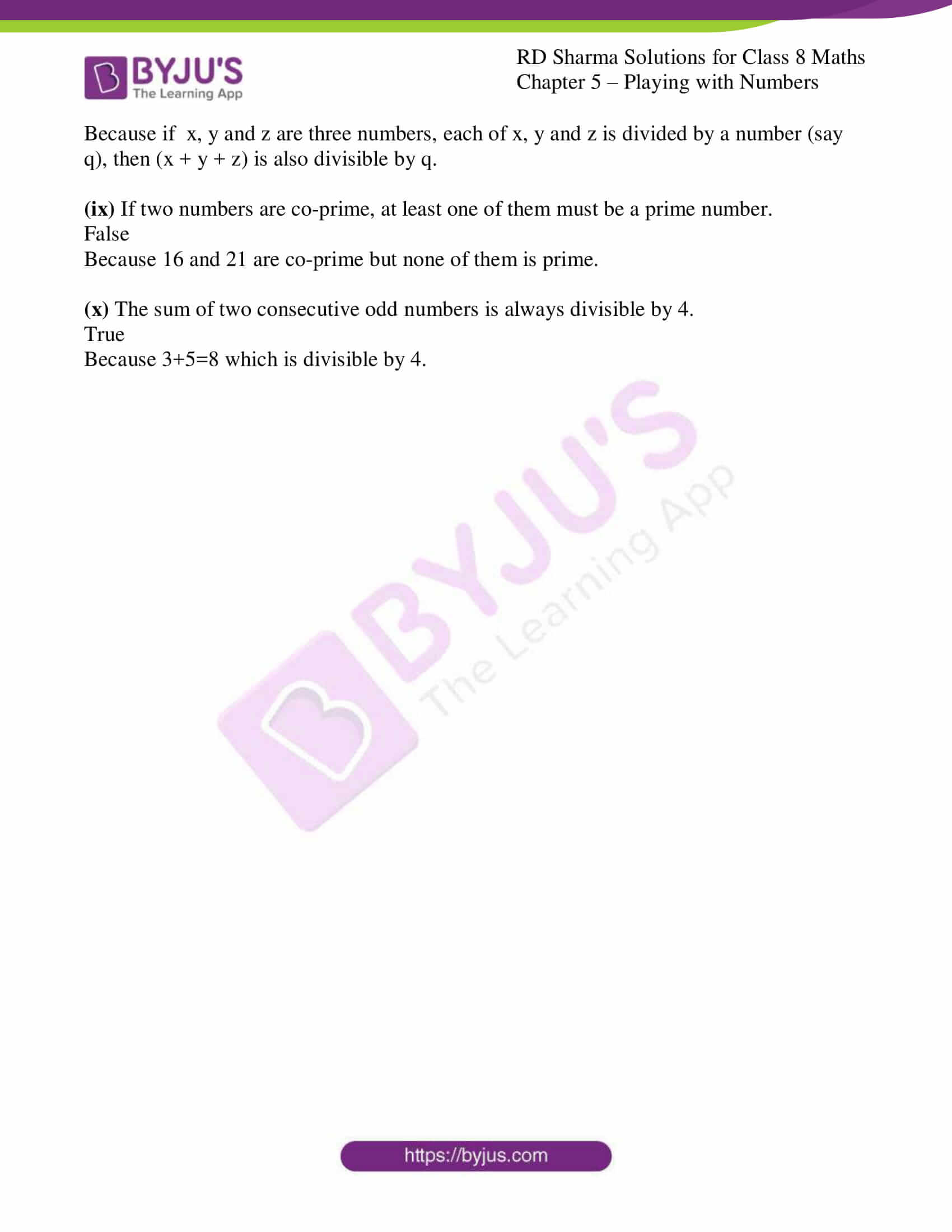### Access answers to Maths RD Sharma Solutions For Class 8 Exercise 5.2 Chapter 5 – Playing with Numbers

1. Given that the number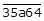is divisible by 3, where a is a digit, what are the possible values of a?

Solution:

We know that the given numberis divisible by 3.

And, if a number is divisible by 3 then sum of digits must be a multiple of 3.

i.e., 3 + 5 + a + 6 + 4 = multiple of 3

a + 18 = 0, 3, 6, 9, 12, 15…..

Here ‘a’ is a digit, where, ‘a’ can have values between 0 and 9.

a + 18 = 18 which gives a = 0.

a + 18 = 21 which gives a = 3.

a + 18 = 24 which gives a = 6.

a + 18 = 27 which gives a = 9.

∴ a = 0, 3, 6, 9

2. If x is a digit such that the number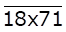is divisible by 3, find possible values of x.

Solution:

We know that the given number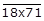is divisible by 3.

And, if a number is divisible by 3 then sum of digits must be a multiple of 3.

i.e., 1 + 8+ x + 7 + 1 = multiple of 3

x + 17 = 0, 3, 6, 9, 12, 15…..

Here ‘x’ is a digit, where, ‘x’ can have values between 0 and 9.

x + 17 = 18 which gives x = 1.

x + 17 = 21 which gives x = 4.

x + 17 = 24 which gives x = 7.

∴ x = 1, 4, 7

3. If x is a digit of the number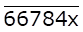such that it is divisible by 9, find possible values of x.

Solution:

We know that the given number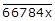is divisible by 9.

And, if a number is divisible by 9 then sum of digits must be a multiple of 9.

i.e., 6 + 6 + 7 + 8 + 4 + x = multiple of 9

x + 31 = 0, 9, 18, 27…..

Here ‘x’ is a digit, where, ‘x’ can have values between 0 and 9.

x + 31 = 36 which gives x = 5.

∴ x = 5

4. Given that the number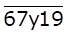is divisible by 9, where y is a digit, what are the possible values of y?

Solution:

We know that the given number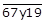is divisible by 9.

And, if a number is divisible by 9 then sum of digits must be a multiple of 9.

i.e., 6 + 7+ y + 1 + 9 = multiple of 9

y + 23 = 0, 9, 18, 27…..

Here ‘y’ is a digit, where, ‘y’ can have values between 0 and 9.

y + 23 = 27 which gives y = 4.

∴ y = 4

5. If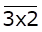is a multiple of 11, where x is a digit, what is the value of x?

Solution:

We know that the given number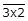is a multiple of 11.

A number is divisible by 11 if and only if the difference between the sum of odd and even place digits is a multiple of 11.

i.e., Sum of even placed digits – Sum of odd placed digits = 0, 11, 22…

x – (3+2) = 0, 11, 22…

x – 5 is a multiple of 11

x – 5 = 0

∴ x = 5

6. If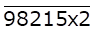is a number with x as its tens digit such that it is divisible by 4. Find all possible values of x.

Solution:

We know that the given number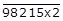is divisible by 4.

A number is divisible by 4 only when the number formed by its digits in unit’s and ten’s place is divisible by 4.

i.e., x2 is divisible by 4

Expanding x2,

10x + 2 = multiple of 4

Here x2 can take values 2, 12, 22, 32, 42, 52, 62, 72, 82, 92

So values 12, 32, 52, 72 and 92 are divisible by 4.

∴ x can take values 1,3,5,7 and 9

7. If x denotes the digit at hundreds place of the number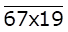such that the number is divisible by 11. Find all possible values of x.

Solution:

We know that the given number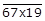is divisible by 11.

A number is divisible by 11 if and only if the difference between the sum of odd and even place digits is a multiple of 11.

i.e., Sum of even placed digits – Sum of odd placed digits = 0, 11, 22…

(6 + x + 9) – (7+1) = 0, 11, 22…

x + 7 is a multiple of 11

x + 7 = 11

∴ x = 4

8. Find the remainder when 981547 is divided by 5. Do that without doing actual division.

Solution:

We know that if a number is divided by 5, then remainder is obtained by dividing just the unit place by 5.

i.e., 7 ÷ 5 gives 2 as a remainder.

∴ Remainder will be 2 when 981547 is divided by 5.

9. Find the remainder when 51439786 is divided by 3. Do that without performing actual division.

Solution:

We know that if a number is divided by 3, then remainder is obtained by dividing sum of digits by 3.

Here, sum of digits (5+1+4+3+9+7+8+6) is 43.

i.e., 43 ÷ 3 gives 1 as a remainder.

∴ Remainder will be 1 when 51439786 is divided by 3.

10. Find the remainder, without performing actual division, when 798 is divided by 11.

Solution:

We know that if a number is divided by 11, then remainder is difference between sum of even and odd digit places.

i.e., Remainder = 7 + 8 – 9 = 6

∴ Remainder will be 6 when 798 is divided by 11.

11. Without performing actual division, find the remainder when 928174653 is divided by 11.

Solution:

We know that if a number is divided by 11, then remainder is difference between sum of even and odd digit places.

i.e., Remainder = 9 + 8 + 7 + 6 + 3 – 2 – 1 – 4 – 5 = 33 – 12 = 21

∴ 21 ÷ 11 gives 10 as remainder

∴ Remainder will be 10 when 928174653 is divided by 11.

12. Given an example of a number which is divisible by
(i) 2 but not by 4.
(ii) 3 but not by 6.
(iii) 4 but not by 8.
(iv) Both 4 and 8 but not by 32

Solution:

(i) 2 but not by 4.

Any number which follows the formula of 4n + 2 is an example of a number divisible by 2 but not by 4.

i.e., 10 where n = 1.

(ii) 3 but not by 6.

Any number which follows the formula of 6n + 3 is an example of a number divisible by 3 but not by 6.

i.e., 15 where n = 1.

(iii) 4 but not by 8.

Any number which follows the formula of 8n + 4 is an example of a number divisible by 4 but not by 8.

i.e., 28 where n = 1.

(iv) Both 4 and 8 but not by 32

Any number which follows the formula of 32n + 8 or 32n + 16 or 32n +24 is an example of a number divisible by both 4 and 8 but not by 32.

i.e., 48 where n = 1.

13. Which of the following statements are true?
(i) If a number is divisible by 3, it must be divisible by 9.
(ii) If a number is divisible by 9, it must by divisible by 3.
(iii) If a number is divisible by 4, it must by divisible by 8.
(iv) If a number is divisible by 8, it must be divisible by 4.
(v) A number is divisible by 18, if it is divisible by both 3 and 6.
(vi) If a number is divisible by both 9 and 10, it must be divisible by 90.
(vii) If a number exactly divides the sum of two numbers, it must exactly divide the numbers separately.
(viii) If a number divides three numbers exactly, it must divide their sum exactly.
(ix) If two numbers are co-prime, at least one of them must be a prime number.
(x) The sum of two consecutive odd numbers is always divisible by 4.

Solution:

(i) If a number is divisible by 3, it must be divisible by 9.

False

Because any number which follows the formula 9n + 3 or 9n + 6 violates the statement.

For example: 6, 12…

(ii) If a number is divisible by 9, it must by divisible by 3.

True

Because 9 is multiple of 3, any number divisible by 9 is also divisible by 3.

(iii) If a number is divisible by 4, it must by divisible by 8.

False

Because any number which follows the formula 8n + 4 violates the statement.

For example: 4, 12, 20….

(iv) If a number is divisible by 8, it must be divisible by 4.

True

Because 8 is multiple of 4, any number divisible by 8 is also divisible by 4.

(v) A number is divisible by 18, if it is divisible by both 3 and 6.

False

Because for example 24, this is divisible by both 3 and 6 but not divisible by 18.

(vi) If a number is divisible by both 9 and 10, it must be divisible by 90.

True

Because 90 is the GCD of 9 and 10, any number divisible by both 9 and 10 is also divisible by 90.

(vii) If a number exactly divides the sum of two numbers, it must exactly divide the numbers separately.

False

Because let us consider an example 6 divide 30, but 6 divides none of 13 and 17 as both are prime numbers.

(viii) If a number divides three numbers exactly, it must divide their sum exactly.

True

Because if x, y and z are three numbers, each of x, y and z is divided by a number (say q), then (x + y + z) is also divisible by q.

(ix) If two numbers are co-prime, at least one of them must be a prime number.

False

Because 16 and 21 are co-prime but none of them is prime.

(x) The sum of two consecutive odd numbers is always divisible by 4.

True

Because 3+5=8 which is divisible by 4.